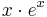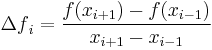# numpy Homework

Implement the following python functions. The name of the exercise should be the name of the function. You will need import numpy! On your own computer I recommend Anaconda because it is installed with numpy by default. Mind that you need the 2.7 version. Or you can use the leibniz.

## matrix (2p)

The function should have 3 parameters, one matrix and two indices:

  M: a matrix
i: a row index
j: a column index


The output should be a matrix (let's call A) with the same size as M. It should be the outer product of the ath row and the ath column of M. In formula:$A_{i,j} = M_{i, b}\cdot M_{a, j}$

You can calculate this kind of product of vectors by multiplying a somethig-by-1 and a 1-by-something matrix.

## derivative (3p)

The function should have 3 parameters, two real numbers and one integer:

  a: the left-hand-side of the interval
b: the right-hand-side of the interval
n: the number of uniformly distributed points in the interval


a < b and n > 1.

• The output should be a numpy vector of length n-2 containing the numerical derivative of the function$x\cdot e^x$ on the given interval, except the endpoints.
• Use the following estimation for the derivative (more about numerical differentiation):${\Delta f}_i = \frac{f(x_{i+1})-f(x_{i-1})}{x_{i+1}-x_{i-1}}$

## Handing-in

Send the exercises as a python code from your math email account to info1hazi@gmail.com

You should attach one python file containing the definition of the required functions without any test code or print command.

The file should be named:

  EN1_HW8_<user account>.py


the subject of the letter should be the same (without the extension). For me example:

  EN1_HW8_borbely.py